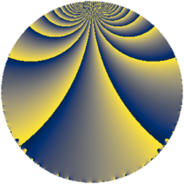# Properties

 Label 108.2.bLevel $108$ Weight $2$ Character orbit 108.b Rep. character $\chi_{108}(107,\cdot)$ Character field $\Q$ Dimension $8$ Newform subspaces $2$ Sturm bound $36$ Trace bound $4$

# Related objects

## Defining parameters

 Level: $$N$$ $$=$$ $$108 = 2^{2} \cdot 3^{3}$$ Weight: $$k$$ $$=$$ $$2$$ Character orbit: $$[\chi]$$ $$=$$ 108.b (of order $$2$$ and degree $$1$$) Character conductor: $$\operatorname{cond}(\chi)$$ $$=$$ $$12$$ Character field: $$\Q$$ Newform subspaces: $$2$$ Sturm bound: $$36$$ Trace bound: $$4$$ Distinguishing $$T_p$$: $$5$$

## Dimensions

The following table gives the dimensions of various subspaces of $$M_{2}(108, [\chi])$$.

Total New Old
Modular forms 24 8 16
Cusp forms 12 8 4
Eisenstein series 12 0 12

## Trace form

 $$8 q + 2 q^{4} + O(q^{10})$$ $$8 q + 2 q^{4} - 2 q^{10} + 4 q^{13} - 22 q^{16} - 18 q^{22} - 12 q^{25} + 18 q^{28} + 28 q^{34} - 20 q^{37} + 22 q^{40} - 16 q^{49} - 8 q^{52} + 4 q^{58} + 28 q^{61} - 10 q^{64} + 54 q^{70} + 16 q^{73} + 36 q^{76} - 56 q^{82} + 8 q^{85} - 18 q^{88} - 36 q^{94} - 8 q^{97} + O(q^{100})$$

## Decomposition of $$S_{2}^{\mathrm{new}}(108, [\chi])$$ into newform subspaces

Label Dim. $$A$$ Field CM Traces $q$-expansion
$$a_2$$ $$a_3$$ $$a_5$$ $$a_7$$
108.2.b.a $$4$$ $$0.862$$ $$\Q(\sqrt{3}, \sqrt{-5})$$ None $$0$$ $$0$$ $$0$$ $$0$$ $$q+\beta _{1}q^{2}+\beta _{2}q^{4}+(\beta _{1}+\beta _{3})q^{5}+(-1+\cdots)q^{7}+\cdots$$
108.2.b.b $$4$$ $$0.862$$ $$\Q(\sqrt{-2}, \sqrt{-3})$$ None $$0$$ $$0$$ $$0$$ $$0$$ $$q+\beta _{1}q^{2}+(1+\beta _{2})q^{4}+(-\beta _{1}-\beta _{3})q^{5}+\cdots$$

## Decomposition of $$S_{2}^{\mathrm{old}}(108, [\chi])$$ into lower level spaces

$$S_{2}^{\mathrm{old}}(108, [\chi]) \cong$$ $$S_{2}^{\mathrm{new}}(36, [\chi])$$$$^{\oplus 2}$$